# Examples using pythagorean identities to simplify trigonometric | Trigonometry | Khan Academy

##By Khan Academy

Examples using pythagorean identities to simplify trigonometric | Trigonometry | Khan Academy

# 10.6 More Angle-Arc Theorems (Practice)

##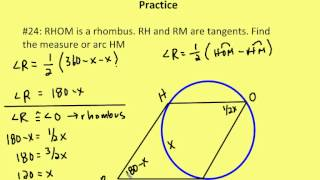By AutenMath

A lesson on 1.) the relationship of inscribed and tangent-chord angles with the same or congruent arcs 2.) the measure of an inscribed angle whose intercepted arc is a semicircle and 3.) the relationship of tangent-tangent angles and their minor arcs

# Part 2 of proof of Heron's formula | Perimeter, area, and volume | Geometry | Khan Academy

##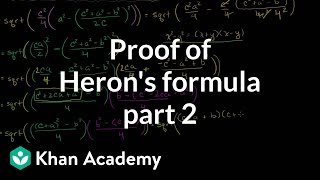By Khan Academy

Video shows that the expression in part 1 is identical to Heron's Formula. This video demonstrates how to use Heron's Formula to determine the area of a triangle while only knowing the lengths of the sides

# 5.7 Proving that Figures are Special Quadrilaterals (Practice)

##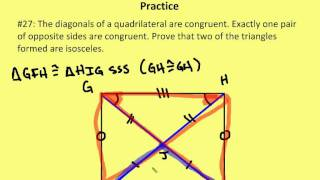By AutenMath

5.7 Proving that Figures are Special Quadrilaterals (Practice)

# Area and Perimeter - Khan Academy

##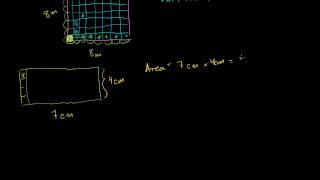By Khan Academy

Start by learning how to calculate the perimeter and area of squares, rectangles, and triangles. Note the explanation of why perimeter measurements are in meters and area measurements are in square meters.

# Area of a Circle (equation derived)

##By Big Bang Physics

The equation for the area of a circle is derived with integration.

# Geometry - Unit 5 Lesson 5 Similarity Transformations

##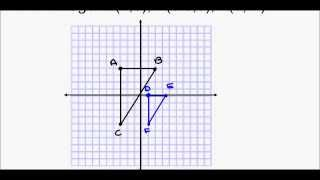By rwalsh213

Geometry - Unit 5 Lesson 5 Similarity Transformations

# 45-45-90 triangles | Right triangles and trigonometry | Geometry | Khan Academy

##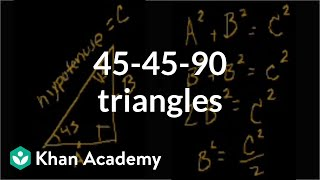By Khan Academy

Video uses an electronic black board with different colored pens. Introduction to 45-45-90 Triangles, these can also be called right triangles because of the 90 degree measurement. These triangles are special because if two angles of a triangle are equal the one side they don’t share is going to be equal. This then goes on to explain Pythagorean Theorem, Side A squared + Side B squared = Side C squared, side C is the hypotenuse or the side that is shared in a triangle. The narrator goes on to explain how to solve, using square roots. This video is for middle school students.

# Basic trigonometry II | Basic trigonometry | Trigonometry | Khan Academy

##By Khan Academy

This video will show you a lot more examples so you can get more comfortable applying the ratios. Remember that this ONLY works for right triangles!

# Significant Digits & Measurements

##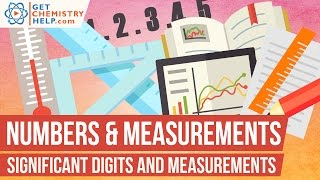By GetChemistryHelp

Significant digits (significant figures, sig figs) indicate the precision of a measurement. This lesson explains how to determine the number of significant digits in a measurement. For practice problems and additional chemistry lessons, visit http://www.getchemistryhelp.com.

...